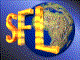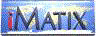| iMatix home page | << | < | > | >>SFLVersion 2.11

### bits_create

```#include "sflbits.h"
BITS *
bits_create (void)
```

#### Synopsis

Creates a new bitstring and initialises all bits to zero. Returns a BITS handle which you should use in all further references to the bitstring.

#### Source Code - (sflbits.c)

```{
BITS
*bits;                          /*  Newly-created bitstring          */
BITBLOCK
*index;                         /*  Newly-created index block        */

bits = mem_alloc (sizeof (BITS));
if (bits)
{
memset (bits, 0, sizeof (BITS));
index = mem_alloc (sizeof (BITBLOCK));
if (index)
{
/*  Set all index fields to 0: bitstring is all zeroes           */
memset (index, 0, sizeof (BITBLOCK));
index-> left       = 0;
index-> right      = 0;
index-> size       = BIT_DATASIZE;
bits-> block    = index;
bits-> block_count = 1;
bits-> free_list   = 0;     /*  No blocks in free list           */
}
else
{
mem_free (bits);
bits = NULL;
}
}
return (bits);
}
```

 | << | < | > | >>Copyright © 1996-2000 iMatix Corporation